After arrays, the second most popular data structure is Linked List. A linked list is a linear data structure, made of a chain of nodes in which each node contains a value and a pointer to the next node in the chain. In this article, let’s see how to implement a linked list in C.

## What is Linked List in C?

A Linked List is a linear data structure. Every linked list has two parts, the data section and the address section that holds the address of the next element in the list, which is called a node.

The size of the linked list is not fixed, and data items can be added at any locations in the list. The disadvantage is that to get to a node, we must traverse to all the way from the first node to the node that we require. The Linked List is like an array but unlike an array, it is not stored sequentially in the memory.

The most popular types of a linked list are:

In the example, the number 43 is present at location 1000 and the address is present at in the previous node. This is how a linked list is represented.

There are multiple functions that can be implemented on the linked list in C. Let’s try to understand them with the help of an example program. First, we create a list, display it, insert at any location, delete a location. The following code will show you how to perform operations on the list.

```#include
#include

void create();
void display();
void insert_begin();
void insert_end();
void insert_pos();
void delete_begin();
void delete_end();
void delete_pos();

struct node
{
int info;
struct node *next;
};
struct node *start=NULL;
int main()
{
int choice;
while(1){

printf("n 1.Create     n");
printf("n 2.Display    n");
printf("n 3.Insert at the beginning    n");
printf("n 4.Insert at the end  n");
printf("n 5.Insert at specified position       n");
printf("n 6.Delete from beginning      n");
printf("n 7.Delete from the end        n");
printf("n 8.Delete from specified position     n");
printf("n 9.Exit       n");
printf("n--------------------------------------n");
scanf("%d",&choice);
switch(choice)
{
case 1:
create();
break;
case 2:
display();
break;
case 3:
insert_begin();
break;
case 4:
insert_end();
break;
case 5:
insert_pos();
break;
case 6:
delete_begin();
break;
case 7:
delete_end();
break;
case 8:
delete_pos();
break;

case 9:
exit(0);
break;

default:
printf("n Wrong Choice:n");
break;
}
}
return 0;
}
void create()
{
struct node *temp,*ptr;
temp=(struct node *)malloc(sizeof(struct node));
if(temp==NULL)
{
printf("nOut of Memory Space:n");
exit(0);
}
printf("nEnter the data value for the node:t");
scanf("%d",&temp->info);
temp->next=NULL;
if(start==NULL)
{
start=temp;
}
else
{
ptr=start;
while(ptr->next!=NULL)
{
ptr=ptr->next;
}
ptr->next=temp;
}
}
void display()
{
struct node *ptr;
if(start==NULL)
{
printf("nList is empty:n");
return;
}
else
{
ptr=start;
printf("nThe List elements are:n");
while(ptr!=NULL)
{
printf("%dt",ptr->info );
ptr=ptr->next ;
}
}
}
void insert_begin()
{
struct node *temp;
temp=(struct node *)malloc(sizeof(struct node));
if(temp==NULL)
{
printf("nOut of Memory Space:n");
return;
}
printf("nEnter the data value for the node:t" );
scanf("%d",&temp->info);
temp->next =NULL;
if(start==NULL)
{
start=temp;
}
else
{
temp->next=start;
start=temp;
}
}
void insert_end()
{
struct node *temp,*ptr;
temp=(struct node *)malloc(sizeof(struct node));
if(temp==NULL)
{
printf("nOut of Memory Space:n");
return;
}
printf("nEnter the data value for the node:t" );
scanf("%d",&temp->info );
temp->next =NULL;
if(start==NULL)
{
start=temp;
}
else
{
ptr=start;
while(ptr->next !=NULL)
{
ptr=ptr->next ;
}
ptr->next =temp;
}
}
void insert_pos()
{
struct node *ptr,*temp;
int i,pos;
temp=(struct node *)malloc(sizeof(struct node));
if(temp==NULL)
{
printf("nOut of Memory Space:n");
return;
}
printf("nEnter the position for the new node to be inserted:t");
scanf("%d",&pos);
printf("nEnter the data value of the node:t");
scanf("%d",&temp->info) ;

temp->next=NULL;
if(pos==0)
{
temp->next=start;
start=temp;
}
else
{
for(i=0,ptr=start;inext;
if(ptr==NULL)
{
return;
}
}
temp->next =ptr->next ;
ptr->next=temp;
}
}
void delete_begin()
{
struct node *ptr;
if(ptr==NULL)
{
printf("nList is Empty:n");
return;
}
else
{
ptr=start;
start=start->next ;
printf("nThe deleted element is :%dt",ptr->info);
free(ptr);
}
}
void delete_end()
{
struct node *temp,*ptr;
if(start==NULL)
{
printf("nList is Empty:");
exit(0);
}
else if(start->next ==NULL)
{
ptr=start;
start=NULL;
printf("nThe deleted element is:%dt",ptr->info);
free(ptr);
}
else
{
ptr=start;
while(ptr->next!=NULL)
{
temp=ptr;
ptr=ptr->next;
}
temp->next=NULL;
printf("nThe deleted element is:%dt",ptr->info);
free(ptr);
}
}
void delete_pos()
{
int i,pos;
struct node *temp,*ptr;
if(start==NULL)
{
printf("nThe List is Empty:n");
exit(0);
}
else
{
printf("nEnter the position of the node to be deleted:t");
scanf("%d",&pos);
if(pos==0)
{
ptr=start;
start=start->next ;
printf("nThe deleted element is:%dt",ptr->info  );
free(ptr);
}
else
{
ptr=start;
for(i=0;inext ;
if(ptr==NULL)
{
return;
}
}
temp->next =ptr->next ;
printf("nThe deleted element is:%dt",ptr->info );
free(ptr);
}
}
}
```

The first part of this code is creating a structure. A linked list structure is created so that it can hold the data and address as we need it. This is done to give the compiler an idea of how the node should be.

```struct node
{
int info;
struct node *next;
};
```

In the structure, we have a data variable called info to hold data and a pointer variable to point at the address. There are various operations that can be done on a linked list, like:

• create()
• display()
• insert_begin()
• insert_end()
• ]insert_pos()
• delete_begin()
• delete_end()
• delete_pos()

These functions are called by the menu-driven main function. In the main function, we take input from the user based on what operation the user wants to do in the program. The input is then sent to the switch case and based on user input.

Based on what input is provided the function will be called. Next, we have different functions that need to be solved. Let’s take a look at each of these functions.

### Create Function

First, there is a create function to create the linked list. This is the basic way the linked list is created. Lets us look at the code.

```void create()
{
struct node *temp,*ptr;

printf("nEnter the data value for the node:t");
scanf("%d",&temp->info);
temp->next=NULL;
if(start==NULL)
{
start=temp;
}
else
{
ptr=start;
while(ptr->next!=NULL)
{
ptr=ptr->next;
}
ptr->next=temp;
}
}

```

Output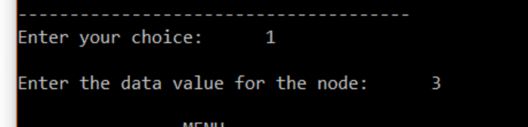First, two pointers are created of the type node, ptr, and temp. We take the value that is needed to be added in the linked list from the user and store it in info part of the temp variable and assign temp of next that is the address part to null. There is a start pointer holding the start of the list. Then we check for the start of the list. If the start of the list is null, then we assign temp to the start pointer. Otherwise, we traverse to the last point where the data has been added.

For this, we assign ptr the start value and traverse till ptr->next= null. We then assign ptr->next the address of temp. In a similar way, there is code given for inserting at the beginning, inserting at the end and inserting at a specified location.

### Display Function

Here’s the code for display function.

```void display()
{
struct node *ptr;
if(start==NULL)
{
printf("nList is empty:n");
return;
}
else
{
ptr=start;
printf("nThe List elements are:n");
while(ptr!=NULL)
{
printf("%dt",ptr->info );
ptr=ptr->next ;
}
}
}
```

Output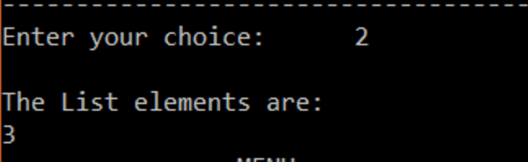In the display function, we first check if the list is empty and return if it is empty. In the next part, we assign the start value to ptr. We then run a loop till ptr is null and print the data element for each node, until ptr is null, which specifies the end of the list.

### Delete Function

Here’ s the snippet of code to delete a node from the linked list.

```void delete_pos()
{
int i,pos;
struct node *temp,*ptr;
if(start==NULL)
{
printf("nThe List is Empty:n");
exit(0);
}
else
{
printf("nEnter the position of the node to be deleted:t");
scanf("%d",&pos);
if(pos==0)
{
ptr=start;
start=start->next ;
printf("nThe deleted element is:%dt",ptr->info  );
free(ptr);
}
else
{
ptr=start;
for(i=0;inext ;
if(ptr==NULL)
{
return;
}
}
temp->next =ptr->next ;
printf("nThe deleted element is:%dt",ptr->info );
free(ptr);
}
}
}
```

Output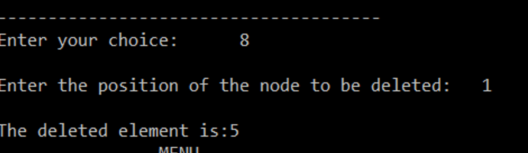In the deletion process, it first checks if the list is empty, if yes it exists. If it is not empty it asks the user for the position to be deleted. Once the user enters the position, it checks if it is the first position, if yes it assigns ptr to start and moves the start pointer to next location and deletes ptr. If the position is not zero, then it runs a for loop from 0 all the way to the pos entered by the user and stored in the pos variable. There is an if statement to decide if the position entered is not present. If ptr is equal to null, then it is not present.

We assign ptr to temp in the for loop, and ptr then moves on to the next part. After this when the position is found. We make the temp variable to hold the value of ptr->next thus skipping the ptr. Then ptr is deleted. Similarly, it can be done for first and the last element deletion.

It is called the doubly linked list because there are two pointers, one point to the next node and other points to the previous node. The operations performed in doubly linked are similar to that of a singly linked list. Here’s the code for basic operations.

```#include
#include
struct Node;
typedef struct Node * PtrToNode;
typedef PtrToNode List;
typedef PtrToNode Position;

struct Node
{
int e;
Position previous;
Position next;
};

void Insert(int x, List l, Position p)
{
Position TmpCell;
TmpCell = (struct Node*) malloc(sizeof(struct Node));
if(TmpCell == NULL)
printf("Memory out of spacen");
else
{
TmpCell->e = x;
TmpCell->previous = p;
TmpCell->next = p->next;
p->next = TmpCell;
}
}

void Delete(int x, List l)
{
Position p, p1, p2;
p = Find(x, l);
if(p != NULL)
{
p1 = p -> previous;
p2 = p -> next;
p1 -> next = p -> next;
if(p2 != NULL)                  // if the node is not the last node
p2 -> previous = p -> previous;
}
else
printf("Element does not exist!!!n");
}

void Display(List l)
{
printf("The list element are :: ");
Position p = l->next;
while(p != NULL)
{
printf("%d -> ", p->e);
p = p->next;
}
}

int main()
{
int x, pos, ch, i;
List l, l1;
l = (struct Node *) malloc(sizeof(struct Node));
l->previous = NULL;
l->next = NULL;
List p = l;
do
{
printf("nn1. CREATEn 2. DELETEn  3. DISPLAYn 4. QUITnnEnter the choice :: ");
scanf("%d", &ch);
switch(ch)
{
case 1:
p = l;
printf("Enter the element to be inserted :: ");
scanf("%d",&x);
printf("Enter the position of the element :: ");
scanf("%d",&pos);
for(i = 1; i < pos; i++) { p = p->next;
}
Insert(x,l,p);
break;

case 2:
p = l;
printf("Enter the element to be deleted :: ");
scanf("%d",&x);
Delete(x,p);
break;

case 3:
Display(l);
break;
}
}
while(ch<4);
}
```

Output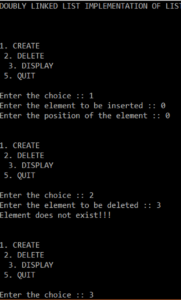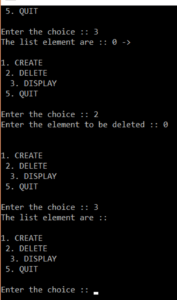So, as you can see the concept of operations is quite simple. The doubly linked list has the same operations as that of singly linked list in C programming language. The only difference is that there is another address variable which help is traversing the list better in a doubly linked list.

I hope you have understood how to perform basic operations on singly and doubly linked list in C.

If you wish to learn Linked List in Java, here’s a complete guide.